# GMAT Math : Interest Problems

## Example Questions

← Previous 1

### Example Question #1 : Interest Problems

Today, I put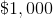in a bank account which promises me a fixed interest rate foryears. How much simple interest (in dollars) have I earned afteryears?

(1) I have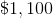in my bank account after year.

(2) The annual interest rate is.

C: BOTH statements TOGETHER are sufficient, but NEITHER statement ALONE is sufficient

B: Statement (2) ALONE is sufficient, but statement (1) ALONE is not sufficient

A: Statement (1) ALONE is sufficient, but statement (2) ALONE is not sufficient

D: EACH statement ALONE is sufficient

E: Statements (1) and (2) TOGETHER are not sufficient

D: EACH statement ALONE is sufficient

Explanation:

From statement (1), we can get the annual interest rate: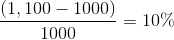.

Therefore after year 2 the simple interest is: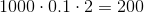. So statement (1) alone is sufficient.

Statement (2) gives us the annual interest rate directly so we can get the result by simply applying the simple interest formula:. So statement (2) alone is sufficient.

Therefore the right answer is D.

### Example Question #2 : Interest Problems

John invests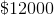in bonds and certificates. The bonds earn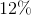interest per year. The certificates earn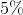interest per year. At the end of the year, John earns a total of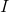as interest. What amount of money did John invest in bonds?

(1) The ratio of interest earned from the certificates to the interest earned from the bonds is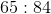.

(2) The total interest earned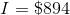.

Statement (2) ALONE is sufficient, but Statement (1) ALONE is not sufficient

Both statements TOGETHER are not sufficient

Both statements TOGETHER are sufficient, but NEITHER statement ALONE is sufficient

Each statement ALONE is sufficient.

Statement (1) ALONE is sufficient, but Statement (2) ALONE is not sufficient

Statement (2) ALONE is sufficient, but Statement (1) ALONE is not sufficient

Explanation:

Statement (1) is not very helpful. Since we do not know the total amount of interest earned or at least the interest earned from one of the financial products (either the bonds or the certificates), we cannot determine the original amount invested in either product.

Using Statement(2), we can set the following equation:

(Let x be the amount invested in bonds)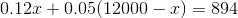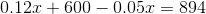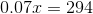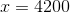John invested $4200 in bonds and$7800 in certificates.

Statement (2) ALONE is sufficient, but Statement (1) ALONE is not sufficient.

### Example Question #3 : Interest Problems

What amount of money did John invest at 8% simple interest?

(1) The amount of interest earned on this investment in one year is $400. (2) John's investment is supposed to accumulate to 7,000 after 5 years. Possible Answers: Statements (1) and (2) TOGETHER are not sufficient. Each Statement ALONE is sufficient. Both statements TOGETHER are sufficient, but NEITHER statement ALONE is sufficient. Statement (2) ALONE is sufficient, but Statement (1) ALONE is not sufficient. Statement (1) ALONE is sufficient, but Statement (2) ALONE is not sufficient. Correct answer: Each Statement ALONE is sufficient. Explanation: (1) The amount of interest earned on this investment in one year is$400.

Let X be the amount invested. The simple annual interest rate is 8%.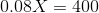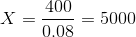The amount of money John invested is $5000. Statement (1) Alone is sufficient. (2) John's investment is supposed to accumulate to 7,000 after 5 years. Let X be the amount invested. The simple annual interest rate is 8%.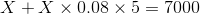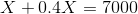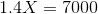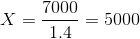The amount of money John invested is$5000. Statement (2) Alone is sufficient.

### Example Question #4 : Interest Problems

Jennifer's grandmother opens up a savings account for her that draws simple interest. No one adds to or withdraws from the account until Jennifer's 18th birthday.

Will there be $10,000 in the account by the time Jennifer turns 18? Statement 1: Jennifer's grandmother opened the account the day Jennifer turned five years old. Statement 2: The account pays 4.5% simple interest. Possible Answers: Statement 1 ALONE is sufficient to answer the question, but Statement 2 ALONE is NOT sufficient to answer the question. EITHER statement ALONE is sufficient to answer the question. BOTH statements TOGETHER are insufficient to answer the question. BOTH statements TOGETHER are sufficient to answer the question, but NEITHER statement ALONE is sufficient to answer the question. Statement 2 ALONE is sufficient to answer the question, but Statement 1 ALONE is NOT sufficient to answer the question. Correct answer: BOTH statements TOGETHER are insufficient to answer the question. Explanation: Simple interest can be calculated using the formula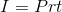whereis the principal, or amount deposited;is the interest rate, expressed as a decimal; andis the time, expressed in years. The question is whether the total of the original principal and the accrued interest will be at least$10,000, or, whether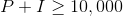or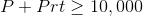is true or false.

Assume both statements. From Statement 1, we get that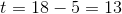, and from Statement 2, we get that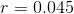. However, neither statement gives the value of principal, so the two statements together are insufficient to answer the question.

### Example Question #5 : Interest Problems

On January 2, Grant uses his $5,000 inheritance to open up a savings account that pays simple interest; he does not add to or withdraw from the account. How many years does it take for the account to be worth$6,000?

Statement 1: The account pays 4.5% annually.

Statement 2: The interest rate of his savings account is 2% less than that of his certificate of deposit in the same bank.

BOTH statements TOGETHER are insufficient to answer the question.

Statement 2 ALONE is sufficient to answer the question, but Statement 1 ALONE is NOT sufficient to answer the question.

BOTH statements TOGETHER are sufficient to answer the question, but NEITHER statement ALONE is sufficient to answer the question.

Statement 1 ALONE is sufficient to answer the question, but Statement 2 ALONE is NOT sufficient to answer the question.

EITHER statement ALONE is sufficient to answer the question.

Statement 1 ALONE is sufficient to answer the question, but Statement 2 ALONE is NOT sufficient to answer the question.

Explanation:

Simple interest can be calculated using the formulawhereis the principal, or amount deposited;is the interest rate, expressed as a decimal; andis the time, expressed in years. Since the main body of the problem gives that Grant deposited $5,000 and that the amount of interest to be earned is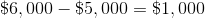, the equation becomes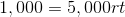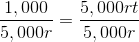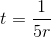Therefore, all that is needed to calculateis the interest rate of the account. Statement 1 gives this explicitly. Statement 2 is irrelevant, as it only compares the interest rate to that of another account, which is not given. ### Example Question #6 : Interest Problems On the same day, Grace and Shannon each open a savings account that draws simple interest. Neither withdraws from or deposits into her account over the next five years. Throughout the five-year period, whose account accrues more interest? Statement 1: Grace's account pays at a rate of 1% per year more than Shannon's account. Statement 2: Grace deposited$5,000 in her account.

BOTH statements TOGETHER are insufficient to answer the question.

Statement 2 ALONE is sufficient to answer the question, but Statement 1 ALONE is NOT sufficient to answer the question.

BOTH statements TOGETHER are sufficient to answer the question, but NEITHER statement ALONE is sufficient to answer the question.

EITHER statement ALONE is sufficient to answer the question.

Statement 1 ALONE is sufficient to answer the question, but Statement 2 ALONE is NOT sufficient to answer the question.

BOTH statements TOGETHER are insufficient to answer the question.

Explanation:

Assume both statements together. If we letbe the interest rate, as a decimal, for Grace's account, the amount of interest Grace's account accrues over five years is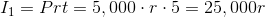The interest rate of Shannon's account is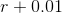, so, if we let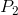be amount she deposited in her account, the amount of interest Shannon's account accrued is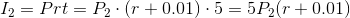Without any information about how much Shannon deposited, or about the actual interest rates, it is impossible to determine which is the greater.

### Example Question #7 : Interest Problems

On the same day, Robin and Rick each open a savings account that draws simple interest. Neither withdraws from or deposits into his or her account over the next five years. Throughout the five-year period, whose account accrues more interest?

Statement 1: Both accounts pay the same interest rate.

Statement 2: Robin deposited $400 more than Rick. Possible Answers: Statement 2 ALONE is sufficient to answer the question, but Statement 1 ALONE is NOT sufficient to answer the question. Statement 1 ALONE is sufficient to answer the question, but Statement 2 ALONE is NOT sufficient to answer the question. BOTH statements TOGETHER are sufficient to answer the question, but NEITHER statement ALONE is sufficient to answer the question. BOTH statements TOGETHER are insufficient to answer the question. EITHER statement ALONE is sufficient to answer the question. Correct answer: BOTH statements TOGETHER are sufficient to answer the question, but NEITHER statement ALONE is sufficient to answer the question. Explanation: Simple interest can be calculated using the formulawhereis the principal, or amount deposited;is the interest rate, expressed as a decimal; andis the time, expresssed in years. For both accounts,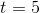, so the amount of interest earned will be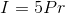Neither statement alone gives sufficient information; Statement 1 alone compares the interest rates but not the amounts of principal, and the reverse holds true for Statement 2. Assume both statements. The accounts pay the same interest rate, which we will continue to call; however, the principal Robin deposited, which we will call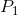, is greater than that deposited by Rick, which we will call. Therefore,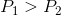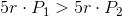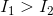That is, Robin's account accrues more interest. ### Example Question #8 : Interest Problems Marshall's grandfather uses$7,000 to open up a savings account for him that draws simple interest. No one adds to or withdraws from the account until Marshall's 21st birthday.

Will there be $10,000 in the account by the time Marshall turns 21? Statement 1: The account pays 3.8% simple interest. Statement 2:Marshall's grandfather opened the account the day Marshall turned six years old. Possible Answers: EITHER statement ALONE is sufficient to answer the question. Statement 2 ALONE is sufficient to answer the question, but Statement 1 ALONE is NOT sufficient to answer the question. Statement 1 ALONE is sufficient to answer the question, but Statement 2 ALONE is NOT sufficient to answer the question. BOTH statements TOGETHER are insufficient to answer the question. BOTH statements TOGETHER are sufficient to answer the question, but NEITHER statement ALONE is sufficient to answer the question. Correct answer: BOTH statements TOGETHER are sufficient to answer the question, but NEITHER statement ALONE is sufficient to answer the question. Explanation: Simple interest can be calculated using the formulawhereis the principal, or amount deposited;is the interest rate, expressed as a decimal; andis the time, expressed in years. The question is whether the total of the original principal and the accrued interest will be at least$10,000. Since this makes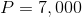, the question becomes whether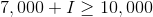or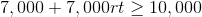is true or false.

Statement 1 and Statement 2 each give the value of only one of the two variables—Statement 1 gives that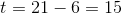and Statement 2 gives that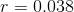—but neither gives the other value. The two together, however, allow us to substitute for both variables and test the inequality for truth: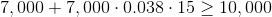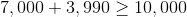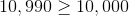This statement is true, so the savings account will indeed accrue to a value above $10,000 by Marshall's 21st birthday. ### Example Question #9 : Interest Problems Jake invests$5000 at 10% simple annual interest and another amount of money atpercent simple interest. What amount of money did Jake invests atpercent interest?

(1) The total amount of interest earned on all investments at the end of one year is $800. (2)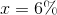. Possible Answers: Statement (2) ALONE is sufficient, but Statement (1) ALONE is not sufficient. Statements (1) and (2) TOGETHER are not sufficient. Statement (1) ALONE is sufficient, but Statement (2) ALONE is not sufficient. Both statements TOGETHER are sufficient, but NEITHER statement ALONE is sufficient. Each Statement ALONE is sufficient. Correct answer: Both statements TOGETHER are sufficient, but NEITHER statement ALONE is sufficient. Explanation: (1) The total amount of interest earned on all investments at the end of one year is$800.

Let A be the amount of money invested at x percent. The total interest earned on both investments is: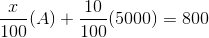We cannot calculate A because x is also unknown. Statement (1) Alone is not sufficient then.

(2) x=6%

Statement (2) Alone is not sufficient.

Combining both statements, we have: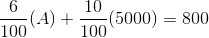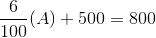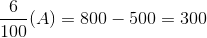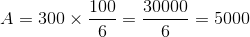Both Statements together are sufficient but neither statement alone is sufficient.

### Example Question #1 : Dsq: Calculating Compound Interest

Jenna needs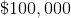years from today to start a business. After talking to an investment banker, Jenna is assured to gain an annually compounded interest of.

What amount of money does Jenna need to invest today?

(1)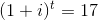(2)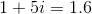Statement (2) ALONE is sufficient, but Statement (1) ALONE is not sufficient

Each statement ALONE is sufficient

Statement (1) ALONE is sufficient, but Statement (2) ALONE is not sufficient

Both statements TOGETHER are sufficient, but NEITHER statement ALONE is sufficient

Both statements TOGETHER are not sufficient.

Statement (2) ALONE is sufficient, but Statement (1) ALONE is not sufficient

Explanation:

Using statement (1), the value of i cannot be determined because t is not specified.

Therefore Statement (1) ALONE is not sufficient.

Using statement (2), the value of i can be determined: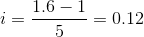We can then set the following equation with X being the amount of money Jenna has to invest today: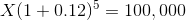We calculate X as follows: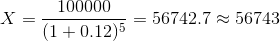← Previous 1

Tired of practice problems?

Try live online GMAT prep today.